Related Articles
Class 9 NCERT Solutions – Chapter 10 Circles – Exercise 10.3
• Last Updated : 04 May, 2021

### Question 1. Draw different pairs of circles. How many points does each pair have in common? What is the maximum number of common points?

Solution:

(i) Two points common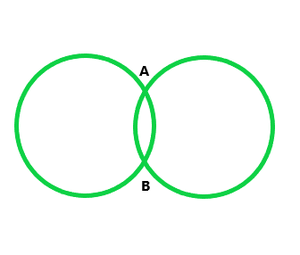(ii) One point common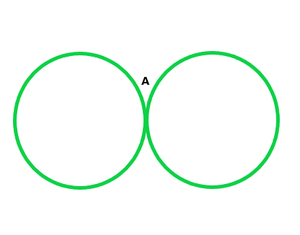(iii) One point common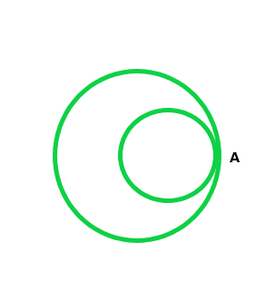(iv) No point common(v) No point commonAs we can analyse from above, two circles can cut each other maximum at two points.

### Question 2. Suppose you are given a circle. Give a construction to find its centre.

Solution:

Let the circle be C1

We need to find its centre.

Step 1: Take points P, Q, R on the circle

Step 2: Join PR and RQ.

We know that perpendicular bisector of a chord passes through centre

So, we construct perpendicular bisectors of PR and RQ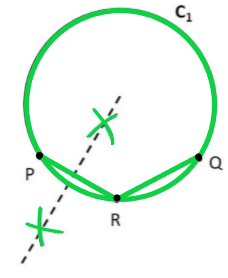Step 3: Take a compass. With point P as pointy end and R as pencil end of the compass, mark an arc above and below PR. Do same with R as pointy end P as pencil end of the compass.

Step 4: Join points intersected by the arcs.

The line formed is the perpendicular bisector of PR.

Step 5: Take compass, with point R as pointy end and Q as pencil end of the compass mark an arc above and below RQ.

Do the same with Q as pointy end and R as pencil end of the compass

Step 6: Join the points intersected by the arcs.

The line formed is the perpendicular bisector of RQ.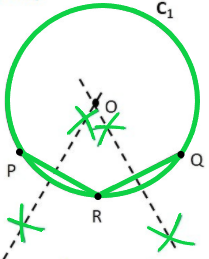Step 7: The point where two perpendicular bisectors intersect is the centre of the circle. Mark it as point O.

Thus, O is the centre of the given circle.

### Question 3: If two circles intersect at two points, prove that their centres lie on the perpendicular bisector of the common chord.

Solution:

Given,

Let circle C1 have centre O and circle C2 have centre X, PQ is th common chord.

To prove: OX is the perpendicular bisector of PQ i.e.

1. PR = RQ

2. ∠PRO = ∠PRX = ∠QRO = ∠QRX = 90°

Construction:

Join PO, PX, QO, QX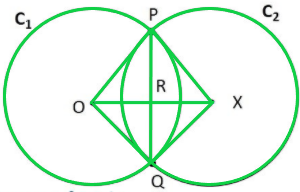Proof:

In △POX and △QOX

OP = OQ     (Radius of circle C1)

XP = XQ     (Radius of circle C2)

OX = OX     (Common)

∴ △POX ≅ △QOX                   (SSS Congruence rule)

∠POX = ∠QOX              (CPCT) —-(1)

Also,

In △POR and △QOR

OP = OQ                              (Radius of circle C1)

∠POR = ∠QOR                 ( From (1))

OR = OR                             (Common)

∴ △OPX ≅ △OQX    (SAS Congruence Rule)

PR = QR                             (CPCT)

& ∠PRO = ∠QRO            (CPCT) —-(2)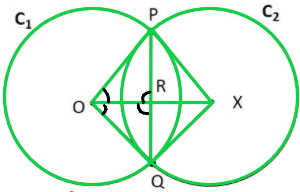Since PQ is a line

∠PRO + ∠QRO = 180°      (Linear Pair)

∠PRO + ∠PRO= 180°          ( From (2))

2∠PRO = 180°

∠PRO = 180° / 2

∠PRO = 90°

Therefore,

∠QRO = ∠PRO = 90°

Also,

∠PRX = ∠QRO = 90°      (Vertically opposite angles)

∠QRX = ∠PRO = 90°      (Vertically opposite angles)

Since, ∠PRO = ∠PRX = ∠QRO = ∠QRX = 90°

∴ OX is the perpendicular bisector of PQ

My Personal Notes arrow_drop_up